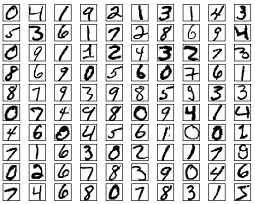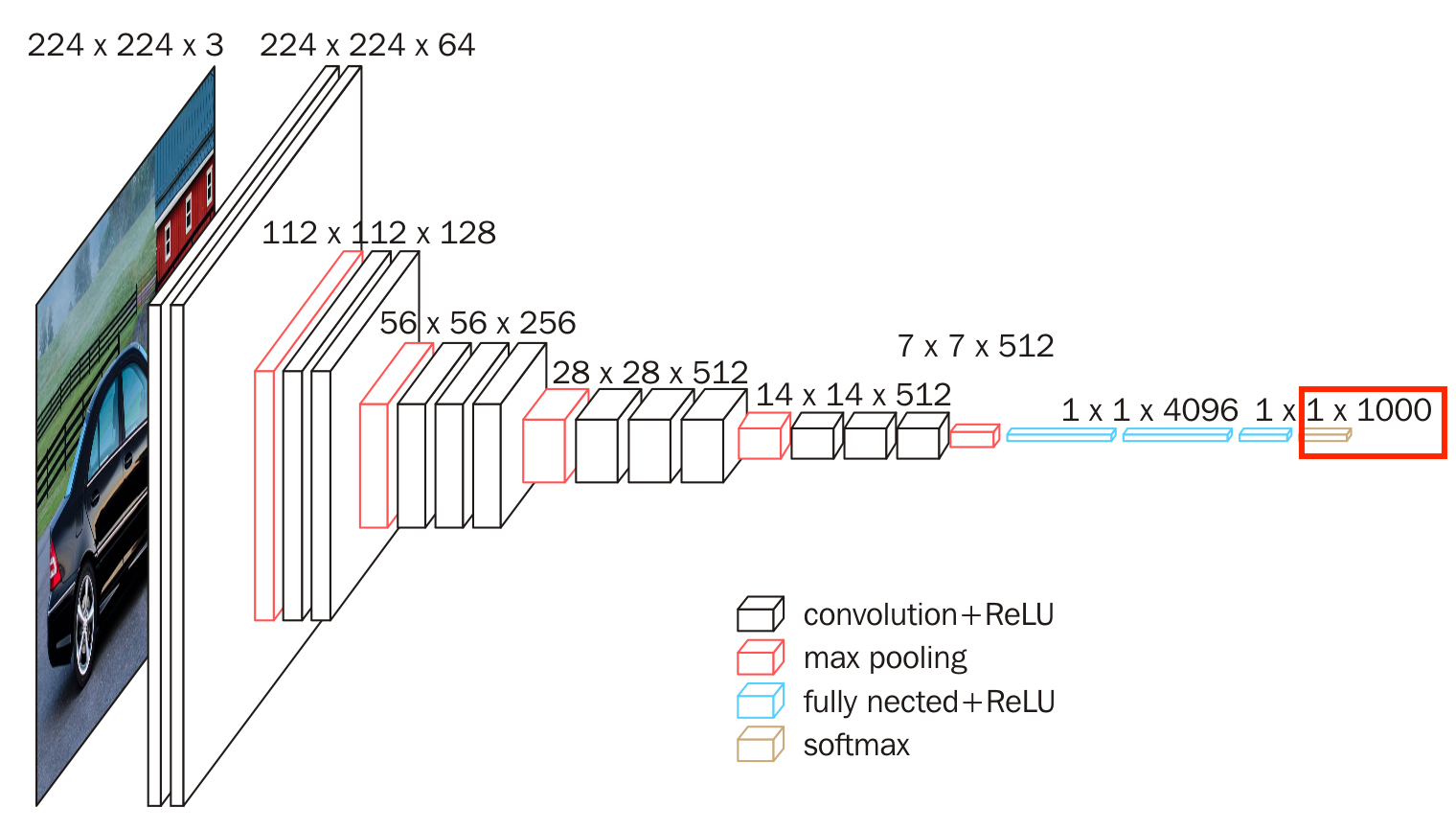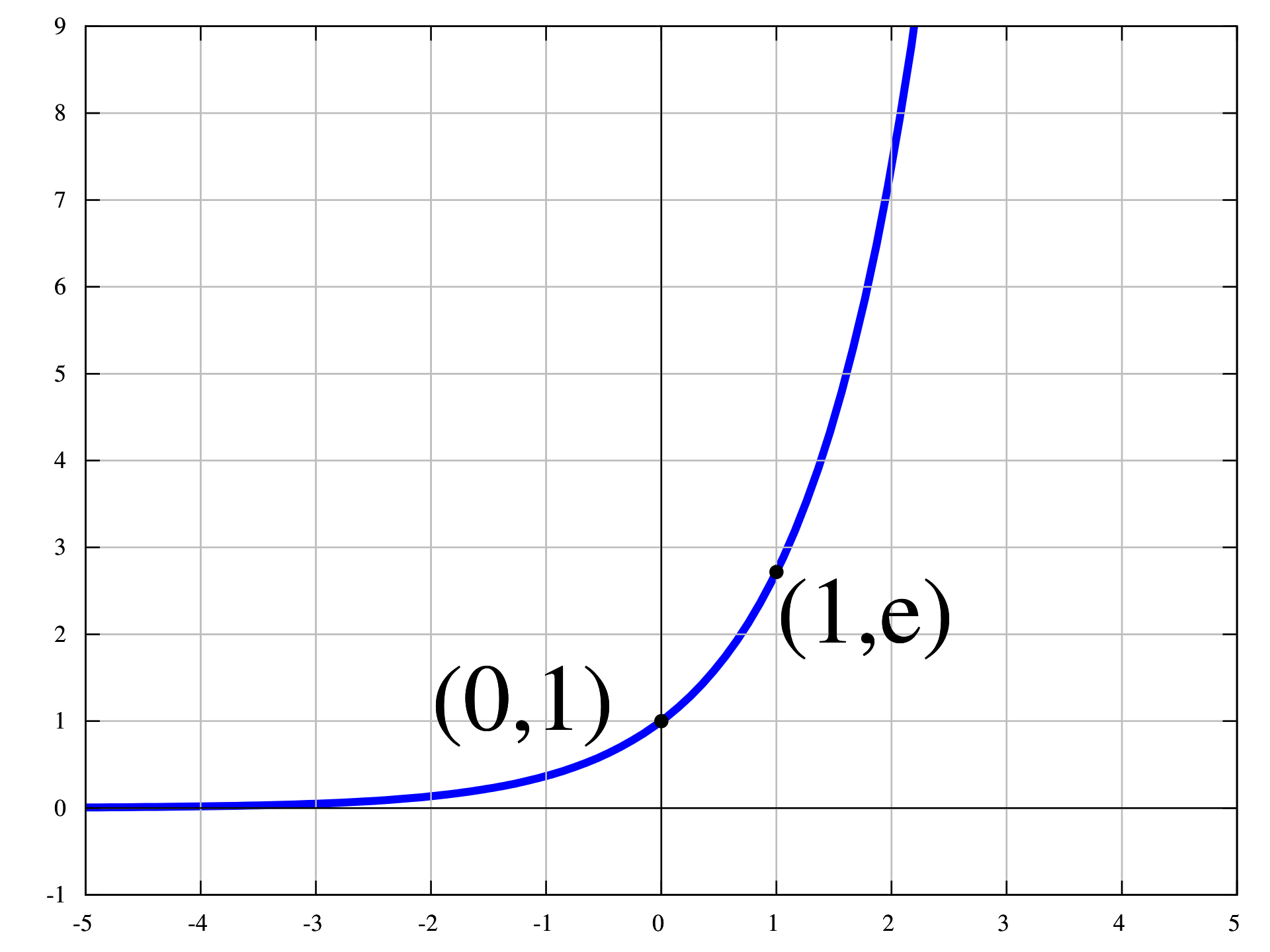# Softmax

Softmax是一种激活函数，它可以将一个数值向量归一化为一个概率分布向量，且各个概率之和为1。Softmax可以用来作为神经网络的最后一层，用于多分类问题的输出。Softmax层常常和交叉熵损失函数一起结合使用。

## 从二分类到多分类#

$g(z)= \frac 1 {1+e^{-z}}$手写体识别数据集mnist

$\text{Softmax}(z_{i}) = \frac{\exp(z_i)}{\sum_j \exp(z_j)}$

Softmax函数可以将上一层的原始数据进行归一化，转化为一个$(0,1)$之间的数值，这些数值可以被当做概率分布，用来作为多分类的目标预测值。Softmax函数一般作为神经网络的最后一层，接受来自上一层网络的输入值，然后将其转化为概率。VGG16是一个图像分类网络，Softmax是VGG16的最后一层，Softmax层的前面是全连接层，Softmax层也是整个VGG16神经网络的输出，输出的是多分类的概率分布

## 指数函数#

Softmax函数使用了指数，对于每个输入$z_i$，需要计算$z_i$的指数。在深度学习进行反向传播时，我们经常需要求导，指数函数求导比较方便：$(e^z)' = e^z$

def softmax(x):    return np.exp(x) / np.sum(np.exp(x), axis=0)

a = np.asarray([2, 3, 5]) softmax(a)array([0.04201007, 0.1141952 , 0.84379473])

def percentile(x):    return x / np.sum(x, axis=0)

percentile(a)array([0.2, 0.3, 0.5])指数函数

b = np.array([20, 300, 5000])softmax(b)

RuntimeWarning: overflow encountered in exp return np.exp(x) / np.sum(np.exp(x), axis=0)

$M = max(z) \\ \text{Softmax}(z_{i}) = \frac{\exp(z_i - M)}{\sum_j \exp(z_j -M)}$